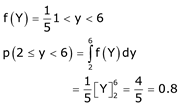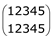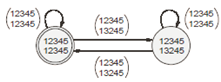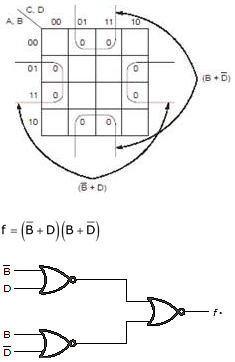# GATE Solved Paper 2017-19 - GATE 2019

• A

4.25• B

4.50• C

4.85• D

5.71• Option : A
• Explanation :
No. of pairs with path length 0=8.0=8.
No. of pairs with path length 1=0.1=0.
No. of pairs with path length 2=8.2=8.
No. of pairs with path length 3=0.3=0.
No. of pairs with path length 4=16.4=16.
No. of pairs with path length 5=0.5=0.
No. of pairs with path length 6=32.6=32.
Total number of possible pairs =8×8=64=8×8=64
So, expected path length,E(x),
=0×864+2×864+4×1664+6×3264=27264=4.25

• A

0.8• B

0.4• C

0.2• D

0.6• Option : A
• Explanation :
It is given that, Polynomial 3x2 + 6xY + 3Y + 6 has only real roots
b2 – 4ax ≥ 0
(6Y)2 – 4(3) (3Y+ 6) ≥ 0
Y2 – Y - 2 ≥ 0<
>Y ∈ (–∞, – 1] ∩ [2, ∞)
⇒ Y ∈ [2, 6)
Since y is uniformly distributed in (1, 6)
Probability distributed function,• A

120• B

125• C

210• D

125• Option : A
• Explanation :
The DFA for accepting L will have 5! = 120 states, since we need one state for every possible permutation function on 5 elements. The starting state will be “id” state, named asand from there
n! arrows will go the n! states each named with a distinct permutation of the set {1, 2, 3, 4, 5}. Since composition of permutation function is closed every arrow has to go to some permutation and hence some state.
Since the language only has those strings where π(x) = id only the starting state (“id” state) will be the final state. Sample machine with only 2 states is shown below• A

3• B

2• C

4• D

5• Option : A
• Explanation :
If a switch has 8 ports then it means it can connect maximum 8 host. and 2 switch can connect 16 hosts independently. but we need one port for both of them to connect with each other. so now one- one port is used from both the switches for connecting then total port available free on both the switch is 7. so maximum 14 host can be connected but for the 15th host we need another switch.
3 switches of ethernet are required to connect 15 computers.
Hence, 3 is correct answer.

• A

3• B

4• C

5• D

6• Option : A
• Explanation :
f = Σm(0, 2, 5, 7, 8, 10, 13, 15)
f = ΠM(1, 3, 4, 6, 9, 11, 12, 14)f can be implemented using 3 NOR gates.
So, option (A) is correct
Related Quiz.
GATE 2019